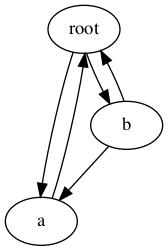copy — Duplicate Objects¶

Purpose: Provides functions for duplicating objects using shallow or deep copy semantics.

The copy module includes two functions, copy() and deepcopy(), for duplicating existing objects.

Shallow Copies¶

The shallow copy created by copy() is a new container populated with references to the contents of the original object. When making a shallow copy of a list object, a new list is constructed and the elements of the original object are appended to it.

copy_shallow.py
import copy
import functools

@functools.total_ordering
class MyClass:

def __init__(self, name):
self.name = name

def __eq__(self, other):
return self.name == other.name

def __gt__(self, other):
return self.name > other.name

a = MyClass('a')
my_list = [a]
dup = copy.copy(my_list)

print('             my_list:', my_list)
print('                 dup:', dup)
print('      dup is my_list:', (dup is my_list))
print('      dup == my_list:', (dup == my_list))
print('dup is my_list:', (dup is my_list))
print('dup == my_list:', (dup == my_list))

For a shallow copy, the MyClass instance is not duplicated, so the reference in the dup list is to the same object that is in my_list.

\$ python3 copy_shallow.py

my_list: [<__main__.MyClass object at 0x101f9c160>]
dup: [<__main__.MyClass object at 0x101f9c160>]
dup is my_list: False
dup == my_list: True
dup is my_list: True
dup == my_list: True

Deep Copies¶

The deep copy created by deepcopy() is a new container populated with copies of the contents of the original object. To make a deep copy of a list, a new list is constructed, the elements of the original list are copied, and then those copies are appended to the new list.

Replacing the call to copy() with deepcopy() makes the difference in the output apparent.

copy_deep.py
import copy
import functools

@functools.total_ordering
class MyClass:

def __init__(self, name):
self.name = name

def __eq__(self, other):
return self.name == other.name

def __gt__(self, other):
return self.name > other.name

a = MyClass('a')
my_list = [a]
dup = copy.deepcopy(my_list)

print('             my_list:', my_list)
print('                 dup:', dup)
print('      dup is my_list:', (dup is my_list))
print('      dup == my_list:', (dup == my_list))
print('dup is my_list:', (dup is my_list))
print('dup == my_list:', (dup == my_list))

The first element of the list is no longer the same object reference, but when the two objects are compared they still evaluate as being equal.

\$ python3 copy_deep.py

my_list: [<__main__.MyClass object at 0x101e9c160>]
dup: [<__main__.MyClass object at 0x1044e1f98>]
dup is my_list: False
dup == my_list: True
dup is my_list: False
dup == my_list: True

Customizing Copy Behavior¶

It is possible to control how copies are made using the __copy__() and __deepcopy__() special methods.

• __copy__() is called without any arguments and should return a shallow copy of the object.
• __deepcopy__() is called with a memo dictionary and should return a deep copy of the object. Any member attributes that need to be deep-copied should be passed to copy.deepcopy(), along with the memo dictionary, to control for recursion. (The memo dictionary is explained in more detail later.)

The following example illustrates how the methods are called.

copy_hooks.py
import copy
import functools

@functools.total_ordering
class MyClass:

def __init__(self, name):
self.name = name

def __eq__(self, other):
return self.name == other.name

def __gt__(self, other):
return self.name > other.name

def __copy__(self):
print('__copy__()')
return MyClass(self.name)

def __deepcopy__(self, memo):
print('__deepcopy__({})'.format(memo))
return MyClass(copy.deepcopy(self.name, memo))

a = MyClass('a')

sc = copy.copy(a)
dc = copy.deepcopy(a)

The memo dictionary is used to keep track of the values that have been copied already, so as to avoid infinite recursion.

\$ python3 copy_hooks.py

__copy__()
__deepcopy__({})

Recursion in Deep Copy¶

To avoid problems with duplicating recursive data structures, deepcopy() uses a dictionary to track objects that have already been copied. This dictionary is passed to the __deepcopy__() method so it can be examined there as well.

The next example shows how an interconnected data structure such as a directed graph can help protect against recursion by implementing a __deepcopy__() method.

copy_recursion.py
import copy

class Graph:

def __init__(self, name, connections):
self.name = name
self.connections = connections

self.connections.append(other)

def __repr__(self):
return 'Graph(name={}, id={})'.format(
self.name, id(self))

def __deepcopy__(self, memo):
print('\nCalling __deepcopy__ for {!r}'.format(self))
if self in memo:
existing = memo.get(self)
return existing
print('  Memo dictionary:')
if memo:
for k, v in memo.items():
print('    {}: {}'.format(k, v))
else:
print('    (empty)')
dup = Graph(copy.deepcopy(self.name, memo), [])
print('  Copying to new object {}'.format(dup))
memo[self] = dup
for c in self.connections:
return dup

root = Graph('root', [])
a = Graph('a', [root])
b = Graph('b', [a, root])

dup = copy.deepcopy(root)

The Graph class includes a few basic directed graph methods. An instance can be initialized with a name and a list of existing nodes to which it is connected. The add_connection() method is used to set up bidirectional connections. It is also used by the deep copy operator.

The __deepcopy__() method prints messages to show how it is called, and manages the memo dictionary contents as needed. Instead of copying the entire connection list wholesale, it creates a new list and appends copies of the individual connections to it. That ensures that the memo dictionary is updated as each new node is duplicated, and it avoids recursion issues or extra copies of nodes. As before, the method returns the copied object when it is done.Deep Copy for an Object Graph with Cycles

The graph shown in the figure includes several cycles, but handling the recursion with the memo dictionary prevents the traversal from causing a stack overflow error. When the root node is copied, it produces the following output.

\$ python3 copy_recursion.py

Calling __deepcopy__ for Graph(name=root, id=4326183824)
Memo dictionary:
(empty)
Copying to new object Graph(name=root, id=4367233208)

Calling __deepcopy__ for Graph(name=a, id=4326186344)
Memo dictionary:
Graph(name=root, id=4326183824): Graph(name=root,
id=4367233208)
Copying to new object Graph(name=a, id=4367234720)

Calling __deepcopy__ for Graph(name=root, id=4326183824)